Courses

# Test: Determinacy & Indeterminacy - 3

## 10 Questions MCQ Test Structural Analysis | Test: Determinacy & Indeterminacy - 3

Description
This mock test of Test: Determinacy & Indeterminacy - 3 for GATE helps you for every GATE entrance exam. This contains 10 Multiple Choice Questions for GATE Test: Determinacy & Indeterminacy - 3 (mcq) to study with solutions a complete question bank. The solved questions answers in this Test: Determinacy & Indeterminacy - 3 quiz give you a good mix of easy questions and tough questions. GATE students definitely take this Test: Determinacy & Indeterminacy - 3 exercise for a better result in the exam. You can find other Test: Determinacy & Indeterminacy - 3 extra questions, long questions & short questions for GATE on EduRev as well by searching above.
QUESTION: 1

### Neglecting axial deformation, the kinematic indeterminacy of the structure shown in the figure below is: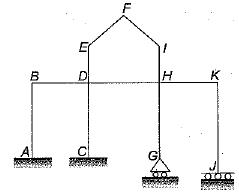Solution:

Kinematic indeterminacy means degree of freedom of structure at various joints.
No rotation or translation is possible at A so degree of freedom at A is zero. There is a possibility of rotation at C but no translation so degree of freedom is one. At G both rotation and translation is possible so. degree of freedom is 2. At J no rotation but translation so d.o.f. is 1 . At B, D, H and K there are 4 rotations and 1 translation so d.o.f. is 5. At E, F and I three rotations and two translations so d.o.f., is 5.
So kinematic indeterminacy,
= 0 + 1 + 2 + 1 + 5 + 5 = 14
Alternate:
From direct formula
External reactions re = 3 + 2 + 1 + 2 = 8
Number of members (m) = 11
Number of rigid joints (j) = 9
Number of hinged joints (j') = 2
There are no internal hinges so number of releases is zero.
rr = 0
Degree of kinematic indeterminacy,
Dk = 3(j + j') - re + r- m
= 3 x (9 + 2) - 8 - 11
= 33 - 19 = 14

QUESTION: 2

### What is the statical indeterminacy for the frame shown below?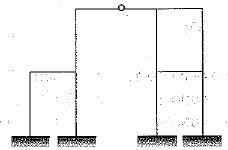Solution:

Static indeterminacy Ds = 3 m + r- rr - 3(j + j') Where,
m = total members = 12
re = total external reactions = 12
j = total number of rigid joints = 11
j' = total number of hybrid joints = 1
rr = total number of released reactions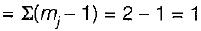∴ Ds = (3 x 12) + 12 - 1 - 3 (11 + 1)
= 36 + 11 - 36 = 11

QUESTION: 3

### The statical indeterminacy for the given 3D frame is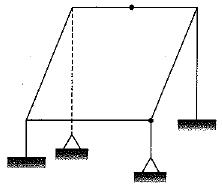Solution:

Ds = Dse + Dsi
Dse = rE - 6
rE = 6 + 3 + 3 + 6 = 18
Dse = 12
Dsi = 6c - rR = 6 x 1 - 3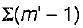= 6 - 3 [(2 - 1) + (3 - 1)]
= 6 - 9 = -3
∴ Ds = 12 - 3 = 9

QUESTION: 4

The statical indeterminacy for the given 2D frame is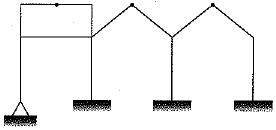Solution:

Ds = Dse + Dsi
Dse = rE - 3
rE = 2 + 3 + 3 + 3 = 11
Dse = 8
Dsi = 3C - r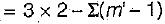= 6 - (2 - 1) + (2 - 1) + (2 - 1) = 3
∴ Ds = 8 + 3 = 11

QUESTION: 5

Consider the following pin-jointed plane frames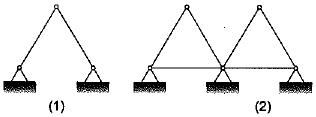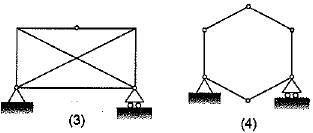Which of these frames are stable?

Solution:
QUESTION: 6

The kinematics indeterminacy of the beam as shown in figure is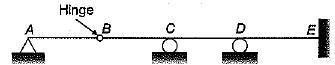Solution:

The kinematic indeterminacy of the beam is given by,
D= 3j - re + rr
Now, j = 5
re = 7
rr = 1
∴ Dk = 3 x 5 - 7 + 1 = 9

QUESTION: 7

The degree of static indeterminacy of the hybrid plane frame as shown in figure is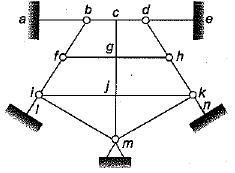Solution:

Ds = Dse + Dsi
Dse = re - 3 = 14 - 3 = 11
Dsi = 3c - rr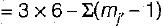= 18 - ( 2 + 2 + 3 + 2 + 3 + 2 + 2)
= 18 - (16) = 2
∴ Ds = 11 + 2 = 13

QUESTION: 8

A plane frame ABCDEFGH shown in figure has clamp support at A hinge supports at G and H, axial force release at C and moment release (hinge) at E. The static (ds) and kinematic (αk) indeterminacies respectively are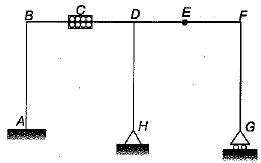Solution:
QUESTION: 9

The total degree of kinematic indeterminacy of the plane frame shown in the given figure considering columns to be axially rigid is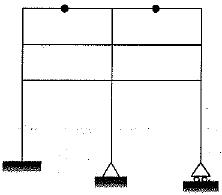Solution:

D= [3(j + j) - re] + rr - m
where,
j = total number of rigid joints = 12
j' = total number of hybrid joints = 2
re = total number of external reactions
= 3+ 2 + 2 = 7
rr =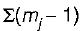= (2 - 1) + (2 - 1) = 2
m = total number of axially rigid member = 9
∴ Dk = [3(12 + 2) - 7] + 2 - 9 = 35 - 7 = 28

QUESTION: 10

The plane pin joint structure shown in figure below is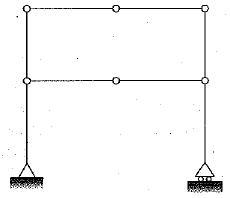Solution: